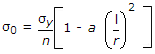# Civil Engineering - Theory of Structures - Discussion

### Discussion :: Theory of Structures - Section 3 (Q.No.25)

25.

For calculating the allowable stress of long columnsis the empirical formula, known as

 [A]. Straight line formula [B]. Parabolic formula [C]. Perry's formula [D]. Rankine's formula.

Explanation:

No answer description available for this question.